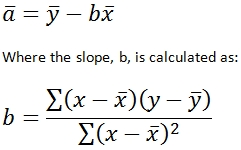# SQL Server TREND function

TREND_q

Updated: 9 August 2010
Use TREND_q to calculate the values along a linear trend. TREND_q fits a straight line (using the method of least squares) to the known-y dataset and the known-x dataset. The equation for TREND_q is:then

TREND = (m * @New_x) + b
Syntax
SELECT [wctStatistics].[wct].[TREND_q] (
<@Known_y_Known_x_RangeQuery, nvarchar(4000),>
,<@New_x, float,>)
Arguments

@Known_y_Known_x_RangeQuery
the select statement, as text, used to determine the known y- and x-values to be used in this function.
@New_x
the new x-value for which you want trend to calculate the y-value. @New_x is an expression of type float or of a type that can be implicitly converted to float.

Remarks
float
Remarks
·         If the number of known-y data points is not equal to the number of known-x data points, TREND_q will return an error.
·         For simpler queries or queries on de-normalized data, use the TREND function.
·         No GROUP BY is required for this function even though it produces aggregated results.
Examples

Using the normalized table #f1, calculate the sales trend for GOOG in 2009, based on data subsequent to the 2003 fiscal year
select 'GOOG' as [Ticker]
,wct.TREND_q(
'SELECT Amount
,FY from #f1 where symbol = ' + char(39) + 'GOOG' + Char(39)
+ ' and DESCR = ' + char(39) + 'Sales' + Char(39)
+ ' and FY > 2003'
,2009) as [2009 Sales]

This produces the following result

Ticker 2009 Sales
------ ----------------------
GOOG   25964.8750000019

(1 row(s) affected)

Using the normalized table #f1, forecast the sales for all the companies in 2009, based on data subsequent to the 2003 fiscal year

select distinct f.symbol as [Ticker]
,wct.TREND_q(
'SELECT Amount
,FY from #f1 where symbol = ' + char(39) + f.symbol + Char(39)
+ ' and DESCR = ' + char(39) + 'Sales' + Char(39)
+ ' and FY > 2003'
,2009) as [2009 Sales]
from #f1 f

This produces the following result

Ticker     2009 Sales
---------- ----------------------
AMZN       21275.0519999992
CSCO       43491.6999999993
EBAY       10099.3569999998
GOOG       25964.8750000019
MSFT       64040.6000000015

(5 row(s) affected)Copyright 2008-2021 Westclintech LLC         Privacy Policy        Terms of Service